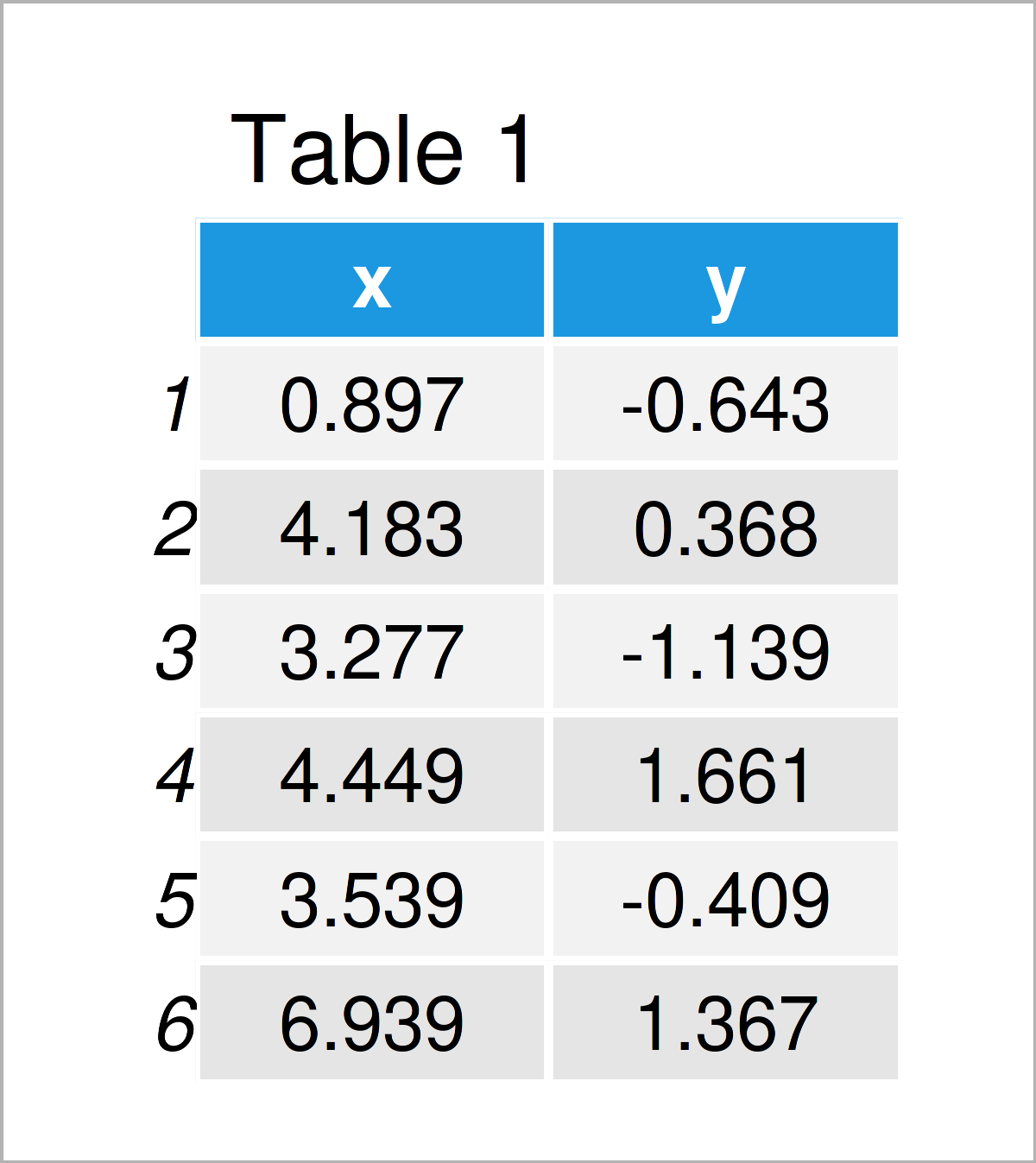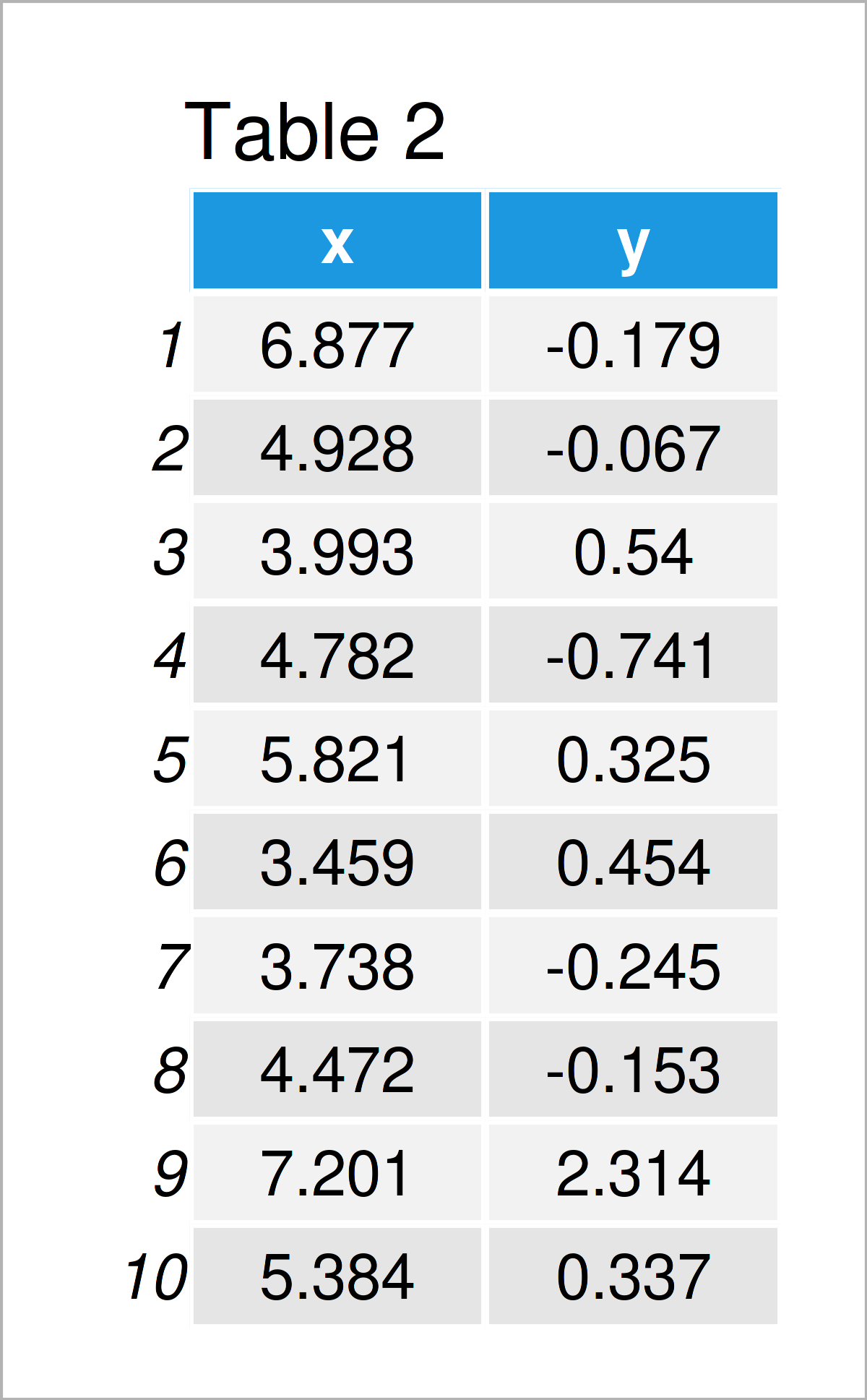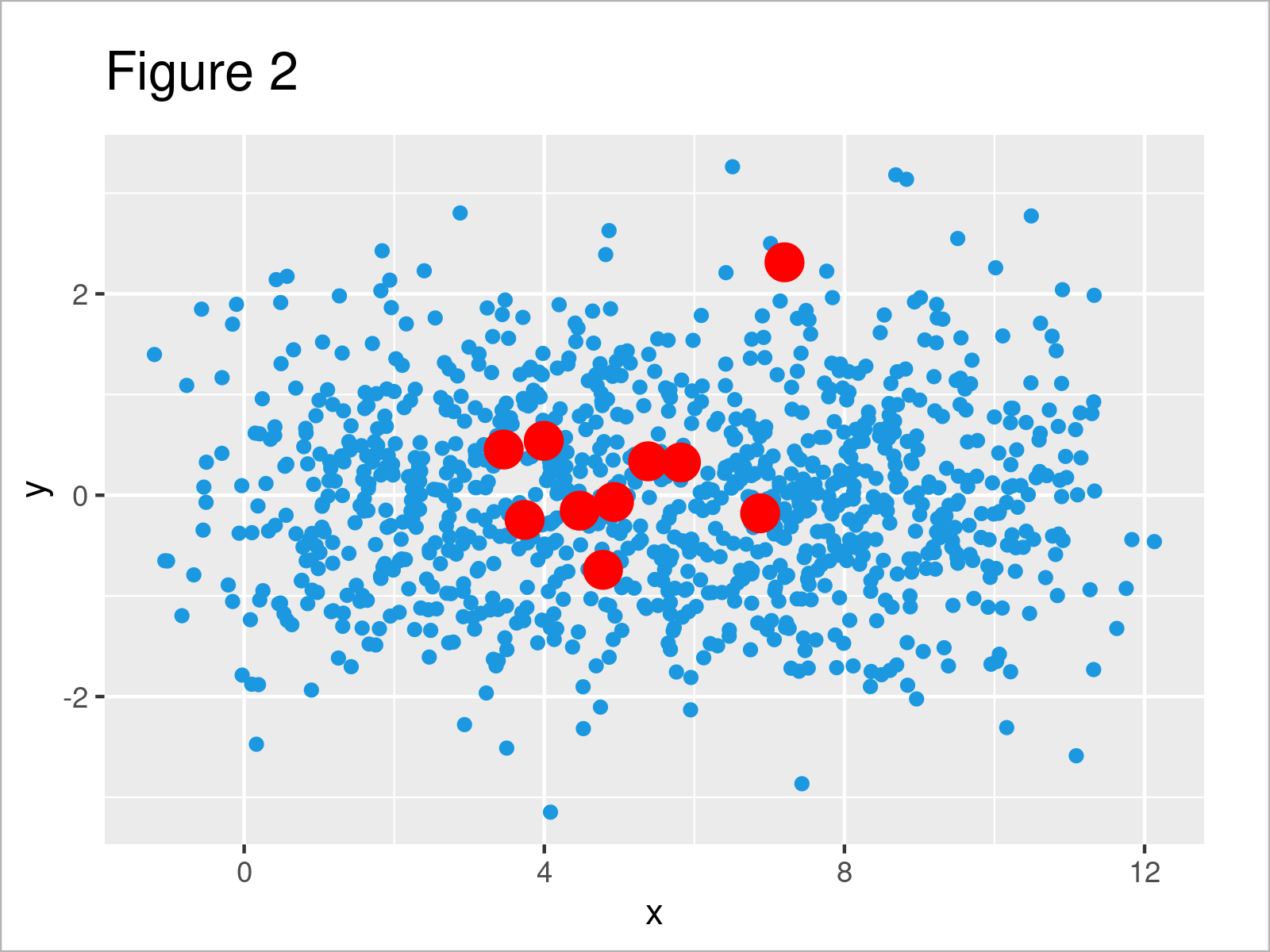# Draw Two Data Sets with Different Sizes in ggplot2 Plot in R (2 Examples)

In this tutorial, I’ll show how to draw a ggplot2 plot of two data frames with different sizes in the R programming language.

The article consists of this content:

Let’s just jump right in.

## Example Data & Software Packages

The following two data sets are used as basement for this R programming tutorial.

First, we have to set a random seed for reproducibility:

`set.seed(328957634)                               # Set seed for reproducibility`

Next, we can use the following R code to create our first example data frame:

```data_large <- data.frame(x = rnorm(1000) + 1:10,  # Create first data frame
y = rnorm(1000))And the following R code to create our second example data frame:

```data_small <- data.frame(x = rnorm(10, 5),        # Create second data frame
y = rnorm(10))
data_small                                        # Print second data frame```After executing the previous R programming codes a larger data frame with 1000 rows and a smaller data frame with only 10 rows have been created.

If we want to plot our example data with the ggplot2 package, we also have to install and load ggplot2:

```install.packages("ggplot2")                       # Install & load ggplot2
library("ggplot2")```

We are set up, let’s move on to the examples!

## Example 1: Draw Two Data Sets with Different Sizes Using ggplot2 Package

This example explains how to draw a ggplot2 plot based on two different data sources.

For this, we have to set the data argument within the ggplot function to be equal to NULL, and then we have to specify the two different data frames within two different calls of a geom_ function (in this case geom_point).

Note that it’s important that both data frames have the same column names.

```ggp1 <- ggplot(NULL, aes(x, y)) +                 # Draw two data frames in ggplot2 plot
geom_point(data = data_large,
col = "#1b98e0") +
geom_point(data = data_small,
col = "red",
size = 5)
ggp1```As shown in Figure 1, we have created a ggplot2 scatterplot based on two different data sets with different sizes.

## Example 2: Combine Two Data Sets Before Drawing in ggplot2 Plot

Another approach is to concatenate both data frames before drawing the in a ggplot2 graphic.

Consider the following R code:

```data_all <- data.frame(rbind(data_large,          # Combine both data frames
data_small),
col = c(rep("#1b98e0", nrow(data_large)),
rep("red", nrow(data_small))),
size = c(rep(1.5, nrow(data_large)),
rep(5, nrow(data_small))))```

After executing the previous syntax, we have created a new data frame that contains the first and second example data frames as well as two additional variables to specify the colors and sizes of our points.

We can now use these data to draw a ggplot2 plot with only one call of the geom_point function.

Note that we have to specify the col and size columns of our new data frame within the geom_point function:

```ggp2 <- ggplot(data_all, aes(x, y)) +             # Draw combined data frame
geom_point(col = data_all\$col,
size = data_all\$size)
ggp2```In Figure 2 it is shown that we have plotted exactly the same graph as in Example 1. However, this time we have merged our data before creating a ggplot2 graphic.

## Video, Further Resources & Summary

Do you need further explanations on the R programming syntax of this article? Then you might want to have a look at the following video on my YouTube channel. In the video, I show the R code of the present page in a live programming session.

Please accept YouTube cookies to play this video. By accepting you will be accessing content from YouTube, a service provided by an external third party.If you accept this notice, your choice will be saved and the page will refresh.

In addition, you may want to have a look at the other articles on my homepage. A selection of articles is listed below.

In summary: In this article, I have demonstrated how to draw a ggplot2 plot of two data frames with different number of rows in R. In case you have any additional questions, please let me know in the comments section.

Subscribe to the Statistics Globe Newsletter

•Nngg
January 14, 2022 10:20 am

Hello,
Thanks for this.

1) Can this be done for ‘boxplots’ instead of points or scatter?

2) What about if there is a 2 more columns:
a) sample-name = where half the names are the same in data_large and data_small, (data_small being a subset of data_large)
b) group = where data_small has 2 groups ‘a’, ‘b’ whilst data_large has 4 groups ‘a’, ‘c’,’d’,’e’

•January 14, 2022 2:53 pm

Hey,

Yes, you can. For instance, you may draw the following plot based on the data created in Example 2:

```ggp2 <- ggplot(data_all, aes(x, fill = col)) +             # Draw combined data frame
geom_boxplot()
ggp2```

Regards,
Joachim